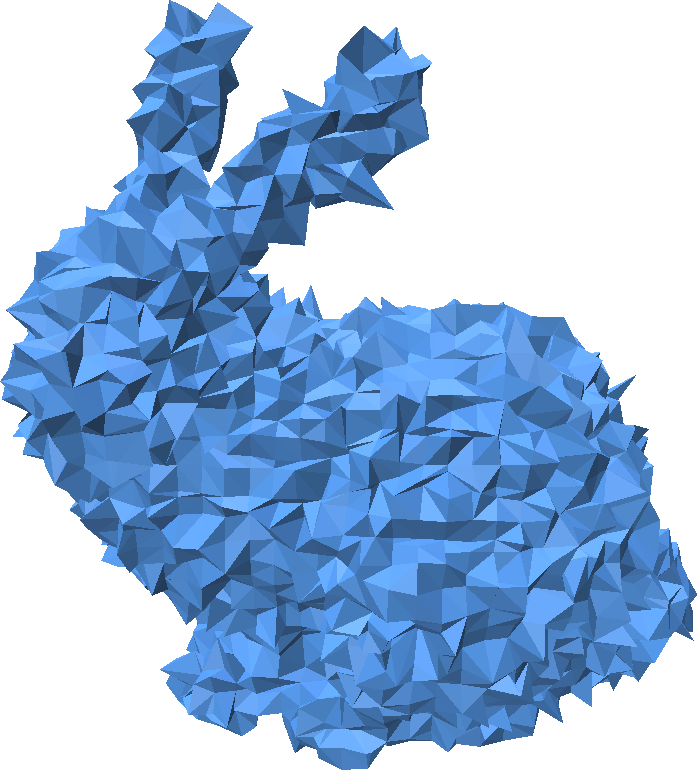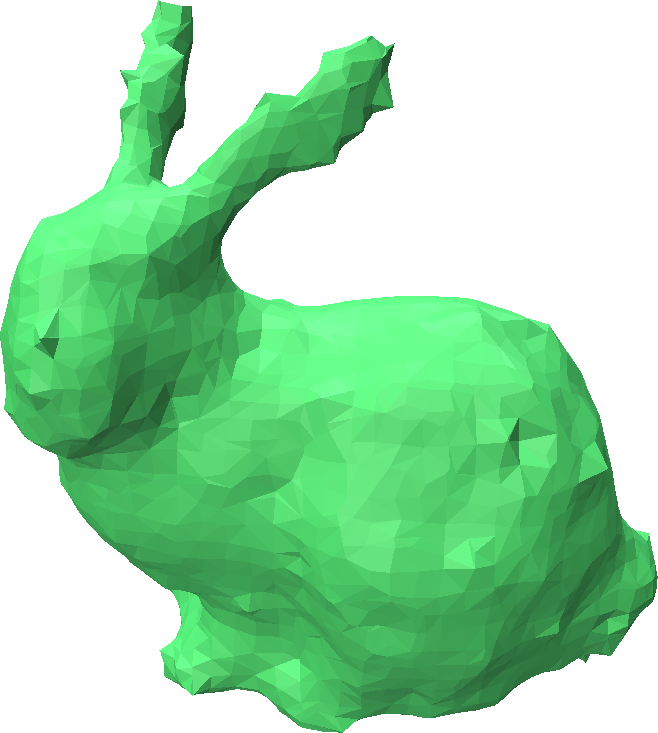# Smoothing a Mesh

Point Cloud Utils supports simple Laplacian Smoothing of a triangle mesh.

import numpy as np
import point_cloud_utils as pcu

n = pcu.estimate_mesh_vertex_normals(v, f)

# Add Gaussian noise to each vertex along its normal
v += np.random.randn(v.shape, 1) * n * 0.02

# Run 4 iterations of Laplacian smoothing. use_cotan_weights is an
# optional parameter specifying whether to use uniform or cotangent
# weights.
# v_smooth has the same shape as v and contains the smooth vertices
v_smooth = pcu.laplacian_smooth_mesh(v, f, num_iters=4, use_cotan_weights=True)